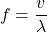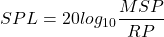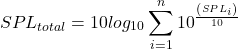# Hz vs dB

#### Engineering

Hz and dB, which represent Hertz and decibels respectively, are units that characterize sound waves. Both units are key in fields such as acoustics, quantum mechanics, and wave mechanics. In this article, you will learn more about Hz and dB, their role in sound perception, as well as review the differences between Hz vs dB.

## What is Hz

Hertz, or Hz, represents the standard units for measuring frequency of oscillations in a wave motion. So, if a wave oscillates 100 times in a second, its frequency is 100Hz. When it comes to sound, frequency relates to the pitch, which describes the lowness or highness of a sound. The higher the frequency, the higher the pitch of the sound. So, sound production from a cymbal is at a higher frequency than what comes from a drum, as the figure below shows.

An average human can detect sounds between 20Hz and 20,000Hz, but this range varies for other animals as the table below highlights.

### Frequency Formula

Clearly, frequency of a wave relates closely to its speed. Mathematically, frequency (f) of a wave is the ratio of its velocity (v) to its wavelength (λ).## What is dB

In simple terms, decibel, or dB, is a measure of a sound’s volume. It characterizes the intensity of a sound and is a function of the amplitude of a sound wave. Generally, a loud sound has a larger pressure variation than a weak sound, and Pascal is the unit of measuring pressure. However, because the human ear detects a very wide range of sound, from 20 × 10-6 Pa to 20 × 102 Pa, and even beyond, it would be inconvenient expressing sound in Pa. So, a logarithmic scale in dB offers an easier way of representing sound pressure level. The dB scale expresses the sound pressure level (SPL) as a ratio of the measured sound pressure (MSP) to the reference pressure (RP) of human hearing, which is 20μPa.Because of the logarithmic scale, the addition of sounds in dB is not straightforward. When aggregating different sounds on a dB scale, the total sound pressure level follows the formula below.## Role of Hz and dB in Sound Perception

Both frequency and amplitude impact the loudness perception when hearing a sound. For example, if a human experiences a sound at 40dB and another at 80dB, the brain interprets the 80dB sound as louder. However, if a human experiences a 60dB tone at 1,500Hz and another tone of 60dB at 15,000Hz, the brain judges the sound at 1,500Hz to be louder. This occurs despite both sounds having the same intensity because human hearing is more sensitive to sounds between 300Hz and 3,000Hz. Moreover, this is the frequency band where most human speech lies, so the ears are less efficient outside these frequencies.

The effect of both amplitude and frequency is used in certain hearing tests. Typically, such tests require a listener to compare tones at different frequencies and adjust the amplitude of one of the tones until both tones sound equally loud. Then, the user’s response is used to produce equal loudness contours, which shows the sensitivity of the ear at varying frequencies. These contours are put on a plot with frequency in Hz on the x-axis, and SPL in dB on the y-axis. The figure below shows an example of this kind of plot.